### 2.3 Magnetic Resonance Imaging

#### 2.3.1 Magnetic Field Gradients

As has been shown in Section 2.2, the fundamental equation of magnetic resonance is the Larmor equation,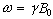. In an NMR experiment a measurement of the frequency of precession of the magnetisation gives information on the field experienced by that group of spins. By manipulating the spatial variation of the field in a known way, this frequency information now yields spatial information.

Consider a linear field gradient in B which increases along the x axis, such that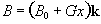(2.36)

where G is the gradient strength. This makes the Larmor equation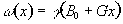(2.37)

or in its more general three dimensional form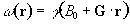(2.38)

Under a linear field gradient along the x axis, all the spins which lie at a particular value of x will precess at the same frequency. The FID from such a sample will contain components from each of the x values represented by the sample, and the frequency spectrum will therefore represent the number of spins that lie along that plane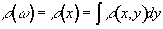(2.39)

as shown in Figure 2.7c.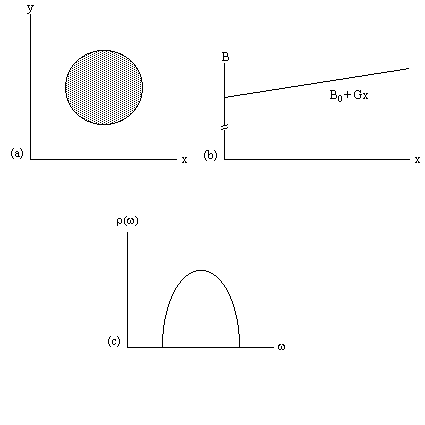##### Figure 2.7. (a) A cylindrical object aligned along the z-axis. (b) Linear field gradient applied along the x-axis. (c) Plot of the number of spins at frequency w

This simple spectrum therefore gives the spatial information about the object being imaged along one dimension. To build up the complete 3D image it is necessary to apply time varying field gradients. A number of methods for doing this are described later in this section, but first the notion of k-space is introduced, which is useful in describing all these techniques.

#### 2.3.2 Reciprocal (k) space

Having denoted the number of spins at a particular location r, as the spin density, r(r), the signal from the sample can be written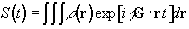(2.40)

The reciprocal space vector is defined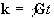(2.41)

and the Fourier relationship between signal and spin density becomes obvious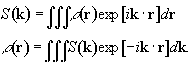(2.41)

Thus k is the conjugate variable for r. The resolution of the image depends on the extent of k-space that is sampled, and it is by looking at how different imaging techniques cover k-space that they can usefully be compared . For example in the previous section a gradient along the x-axis was applied to a cylindrical sample. This meant that values in kx could be sampled, but not in ky and so the complete 2D structure could not be obtained.

There are imaging techniques which sample all three dimensions of k space, but most techniques reduce the problem to two dimensions by applying slice selection.

#### 2.3.3 Slice Selection

Slice selection is a technique to isolate a single plane in the object being imaged, by only exciting the spins in that plane. To do this an r.f. pulse which only affects a limited part of the NMR spectrum is applied, in the presence of a linear field gradient along the direction along which the slice is to be selected (Figure 2.8). This results in the excitation of only those spins whose Larmor frequency, which is dictated by their position, is the same as the frequency of the applied r.f. pulse.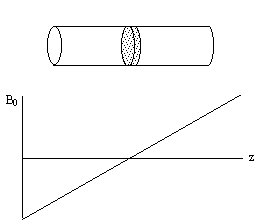##### Figure 2.8. A long cylindrical object aligned along the z-axis in a field gradient which increases linearly with increasing z.

Consider an r.f. pulse of duration 2t which is applied in the presence of a gradient along some axis, say z, such that 2tgB1= p/2 (a 90 degree pulse). The spins where B=B0 (i.e. z=0) will precess into the transverse plane, whilst those where B>>B0 (i.e. z>>0) will precess about Beff=Dw/g k+B1i, and have little effect on the transverse magnetisation (Figure 2.9).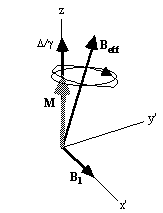##### Figure 2.9. Effect of a 90 degree pulse on (a) off-resonant spins and (b) near-resonant spins.

To tailor the shape of slice selected it is necessary to modulate the pulse profile. For small flip angles the relationship between the pulse modulation and slice profile can be derived. To do this consider applying an r.f. pulse lasting from t=-T to +T, to a sample in a gradient Gz. The Bloch equations as shown earlier (equation. 2.29) in the rotating frame, neglecting T1 and T2 relaxation, become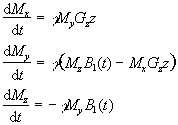(2.43)

Now these are transformed to another frame of reference which rotates at angular frequency gGzz. Each slice will have a different reference frame, depending on the value of z, but considering just one of these gives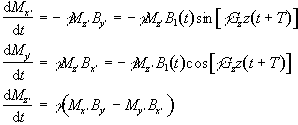(2.44)

with the two reference frames coinciding at t=-T. If the assumption is made that the flip angles are small then it is possible to say that Mz' varies very little, and takes the value M0. So equations 2.44 become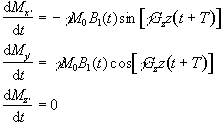(2.45)

If Mx and My are treated as real and imaginary parts of the complex magnetisation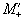, the equation of motion becomes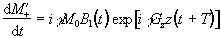(2.46)

By integrating and converting back to the original (rotating) frame of reference, since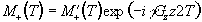gives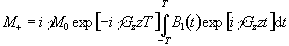(2.47)

This is the Fourier transform of the r.f. pulse profile, so a long pulse gives a narrow slice and vice-versa. This equation also shows one other thing about the magnetisation in the slice after the pulse. There is a phase shift through the slice of gGzzT which will cause problems for imaging later. This can be removed by reversing the gradient Gz for a duration T, which is known as slice refocusing.

To excite a sharp edged slice, the r.f. pulse modulation required is a sinc function. For practical implementation of this kind of pulse it is necessary to limit the length of the pulse, and so usually a five lobe sinc function is used. The above approximation is only correct for small pulse angles. For larger flip angles, it is hard to analytically determine the pulse modulation required for a desired slice profile, and so it is usually necessary to use some form of iterative method to optimise pulses . Using such techniques it is possible to design a range of pulses which are able to select different slice profiles and carry out other spin manipulation techniques such as fat or water suppression. It is also possible to design pulses which do not require refocusing . Usually the limit in such novel designs is their practical implementation, and so the most common three pulse modulations used are a 'hard' pulse, with top-hat modulation, a Gaussian modulation or a 'soft' pulse, with truncated sinc modulation.

If any slice other than the central (B=B0) slice is required, then the frequency of oscillation can be altered and the slice selected will be at the position along the z-axis given by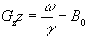(2.48)

#### 2.3.4 Early MR Imaging Techniques

The extensively used techniques in MRI are all Fourier based, that is the 'spin-warp' technique and Echo Planar Imaging (EPI) . However the early MR images used point and line methods and these are described here, along with the technique of projection reconstruction.

The f.o.n.a.r. technique (field focused nuclear magnetic resonance) was proposed by Damadian, and was used to produce the first whole body image in 1977 . The basis of the technique is to create a shaped B0 field which has a central homogeneous region, surrounded by a largely inhomogeneous region. The signals from the inhomogeneous regions will have a very short T2* and can thus be distinguished from the signal from the homogeneous region. By scanning the homogeneous field region across the whole of the sample an image can be made up. This is a time consuming procedure taking tens of minutes for a single slice. The two advantages of the technique are its conceptual simplicity and the lack of a requirement for the static field to be homogeneous over a large area. Shaped r.f. pulses can also be used to isolate a small region, and such methods form the basis of localised spectroscopy.

As was shown in section 2.3.1, if we have only a one dimensional object then a single linear gradient is sufficient to locate position directly from the FID by Fourier transformation. If then the three dimensional sample can be reduced to a set of one dimensional components then the whole sample can be imaged. This can be done by selective irradiation, or slice selection, in two of the dimensions. First, in the presence of a gradient along the z-axis, a selective pulse is applied which saturates all the spins outside the plane of interest (Figure 2.10a). Then a gradient is applied along the x-axis, and those spins not saturated are tipped into the transverse plane by a selective 90 degree pulse (Figure 2.10b). Immediately after the second r.f pulse the only region with any coherent transverse magnetisation is the line of intersection of the two selected planes. A gradient is now applied along the y-axis, and the evolution of the FID recorded. Fourier transform of the FID gives the proton densities along that line. By repeating the line selection for all the lines in the plane, an image of the whole of the plane can be built up. There are many variations of the line scan technique, some of which utilise 180 degree pulses, but they are inefficient in comparison to the Fourier methods discussed in the next section.

(a)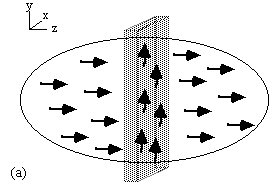(b)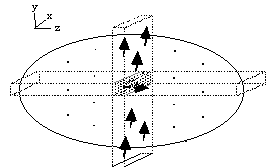##### Figure 2.10. Line selection using two rf pulses. (a) In the presence of a gradient along the z-axis, all spins outside the shaded plane are saturated. (b) In the presence of a gradient along the x-axis those spins not saturated in the selected horizontal plane are tipped into the transverse plane and can be observed in the presence of a gradient along the y-axis

One final method of interest is projection reconstruction. This is the method used to build up X-ray CT scans , and was the method used to acquire the early MR images. Following slice selection, a gradient is applied along the x-axis and the projections of the spin densities onto that axis obtained by Fourier transformation of the FID. Then a linear gradient is applied along an axis at some angle to the x-axis, q. This can be achieved by using a combination of the x and y gradients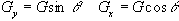.

(2.49)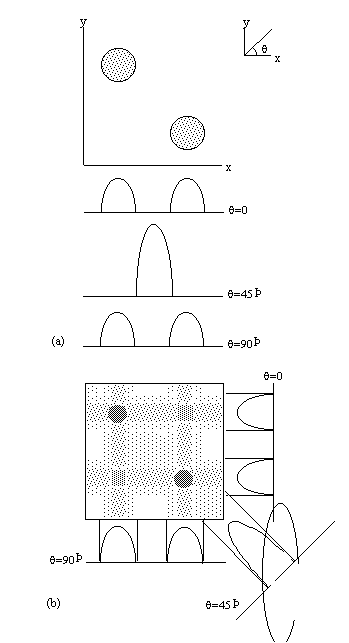##### Figure 2.11. The technique of Projection Reconstruction. (a) Two cylindrical objects are placed in the x-y plane and their projection onto three axes, angled at 0, 45 and 90 degrees to the x-axis are recorded, (b) By back projecting the projections, the location of the objects can be ascertained.

Projections are taken as q is incremented up to 180 degrees (Figure 2.11a). The set of projections can then be put together using back projection. This distributes the measured spin densities evenly along the line normal to the axis it was acquired on. By reconstructing all the angled projections the image appears. There is however a blurred artefact across the whole image, where an attempt was made to assign spin density to areas where there is in fact none, and star artefacts where a finite number of projections have been used to define point structures. This can be corrected using a technique called filtered back projection, which convolves each of the profiles with a filter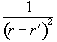(2.50)

This is done in the Fourier domain by dividing the Fourier projections by the modulus of the vector k as defined in section 2.3.2.

Projection reconstruction has been largely superseded by methods which sample k-space more uniformly.

#### 2.3.5 Fourier and Echo Planar Imaging

Quadrature detection of the FID means that the phase as well as the frequency of the signal can be recorded. This is utilised in the Fourier techniques described in this section.

The 'spin-warp' method (often called 2DFT) as described by Edelstein et. al.  is a development of the earlier technique of Fourier zeugmatography proposed by Kumar, Welti, and Ernst . The Fourier zeugmatography sequence can be split into three distinct sections, namely slice selection, phase encoding, and readout. The pulse sequence diagram for the sequence is shown in Figure 2.12. Such diagrams are commonly used to describe the implementation of a particular MR sequence, and show the waveform of the signal sent to the three orthogonal gradient coils and the r.f. coil.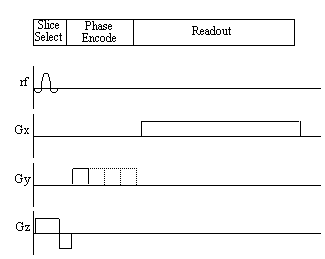##### Figure 2.12. Pulse sequence diagram for the Fourier zeugmatography imaging technique.

Having excited only those spins which lie in one plane, a gradient is applied along the y-axis. This will cause the spins to precess at a frequency determined by their y position, and is called phase encoding. Next a gradient is applied along the x-axis and the FID is collected. The frequency components of the FID give information of the x-position and the phase values give information of the y-position.

More specifically, if a gradient of strength Gy is applied for a time ty during the phase encoding stage, and then a gradient Gx is applied for a duration tx the signal recorded in the FID is given by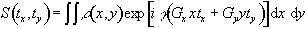(2.51)

By writing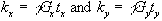equation (2.51) becomes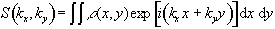(2.52)

so the single step is equivalent to sampling one line in the kx direction of k-space. To cover the whole of k-space it is necessary to repeat the sequence with slightly longer periods of phase encoding each time (Figure 2.13).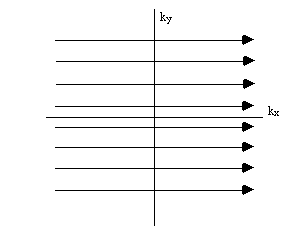##### Figure 2.13. K-space sampling with Fourier zeugmatography

Having acquired data for all values of kx and ky, a 2D Fourier transform recovers the spin density function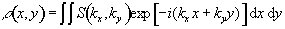(2.53)

Figure 2.14 illustrates how the magnetisation evolves under these two gradients.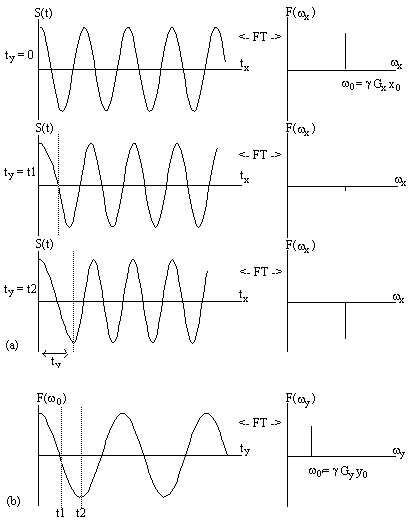##### Figure 2.14. Two dimensional Fourier image reconstruction following Fourier zeugmatography. (a) A single magnetisation vector at position (x0, y0), is allowed to evolve under a gradient along the y axis for a time ty, before being observed under an x gradient. The real components of the Fourier transform of the FID's are shown, giving the x position. (b) Having acquired FID's for all values of ty, a Fourier transform along the second dimension gives the y position.

One drawback of this technique is that the time between exciting the spins, and recording the FID varies throughout the experiment. This means that the different lines in the ky direction will have different weighting from T2* magnetisation decay. This is overcome in spin-warp imaging by keeping the length of the y gradient constant for each acquisition, and varying ky by changing the gradient strength. The pulse diagram for this technique is shown in Figure 2.15.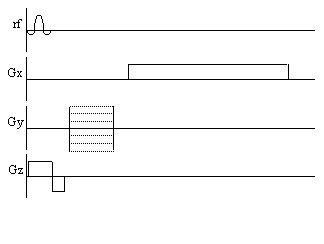##### Figure 2.15. Pulse sequence diagram for the spin-warp technique. Phase encoding is performed by varying the magnitude of the y gradient.

It is desirable to have as much signal as possible for each FID, and a necessity that the amount of transverse magnetisation available immediately after the r.f. pulse is the same for each line. This can be a problem since the recovery of longitudinal magnetisation is dependent on spin-lattice relaxation, and T1 values in biomedical imaging are of the order of seconds. Keeping the time between adjacent spin excitations, often known as TR, the same throughout the image acquisition will keep the transverse magnetisation the same for each FID, provided the first few samples are discarded to allow the system to come to a steady state. Leaving the magnetisation to recover fully, however, would be very costly in time, so it is usually necessary to have a TR which is less than T1. To maximise the signal received for small TR values it is possible to use a smaller flip angle than 90 degrees. The transverse magnetisation that is available after such a pulse is less than it would be after a 90 degree pulse, but there is more longitudinal magnetisation available prior to the pulse. To optimise the flip angle q, for a particular TR, we first assume that the steady state has been reached, that is that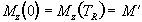(2.54)

Now the magnetisation is flipped by a q degree pulse, and the z-magnetisation becomes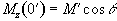.

(2.55)

The recovery of the magnetisation is governed by the equation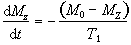(2.56)

which can be integrated to find M',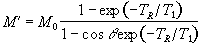(2.57)

The transverse magnetisation following the pulse, which we want to maximise, is given by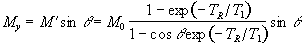(2.58)

which has its maximum value when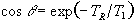(2.59)

The angle this occurs at is known as the Ernst angle . The amount of signal available is very dependent on the repetition time TR. For example, if a sample has a T1 of 1s, then at a TR of 4s M'=0.98M0, however as TR is reduced to 500ms, M'=0.62M0.

If a 3D volume is to be imaged then it is possible to acquire extra slices with no time penalty. This is because it is possible to excite a separate slice, and acquire one line of k-space, whilst waiting for the longitudinal magnetisation of the previous slice to recover. This technique is called multi-slicing.

The common implementation of the spin-warp technique, FLASH (Fast Low-Angle SHot imaging) , uses very small flip angles (~5 degrees) to run at with a fast repetition rate, acquiring an entire image in the order of seconds.

In Echo Planar Imaging [18, 19] (EPI) the whole of k-space is acquired from one FID. This is possible because, having acquired one set of frequency information, the sign of the readout gradients can be reversed and the spins will precess in the opposite direction in the rotating frame (Figure 2.16) and subsequently rephase causing a regrowth of the NMR signal. This is a called a gradient echo. By switching the readout gradient rapidly, the whole of k-space can be sampled before spin-spin (T2) relaxation attenuates the transverse magnetisation. Phase encoding is again used in order to sample ky.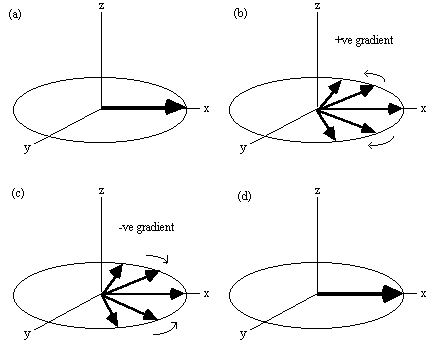##### Figure 2.16. Evolution of the magnetisation vector in the rotating frame, under a gradient echo (assuming no T2 relaxation). (a) Immediately after a 90 degree pulse all the spins are in phase. (b) A gradient will increase the precession frequency of some spins and reduce that of others. (c) Reversal of the gradient at time T causes the spins to refocus, (d) coming back to their initial state at time 2T.
The pulse sequence diagrams and k-space trajectories for EPI are shown in Figure 2.17.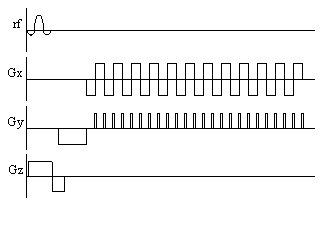##### Figure 2.17. (a) Pulse sequence diagram and (b) k-space sampling pattern for echo planar imaging

The three gradients in EPI are usually labelled the slice select (z), blipped (y) and switched (x), because of their respective waveforms. Echo planar imaging is a technically demanding form of MRI, usually requiring specialised hardware, however it has the advantage of being a very rapid imaging technique, capable of capturing moving organs like the heart, and dynamically imaging brain activation. This is only a brief introduction to EPI, since its strengths and limitations are discussed in more detail in the following chapters.

#### 2.3.6 Other Imaging Sequences

There are numerous variations on the basic MRI sequences described above. Several of them, notably interleaved EPI, are explained in other chapters. Other important sequences are outlined here.

It was shown in the previous section how phase encoding enabled the information on the second dimension to be added to the one dimensional line profile. It is possible to extrapolate this procedure to the third dimension by introducing phase encoding along the z axis. Thus the 2D-FT technique is extended to a 3D-FT technique . All such volumar imaging sequences first involve the selection of a thick slice, or slab. Then phase encoding is applied in the z-direction and the y-direction, followed by a readout gradient in the x-direction, during which the FID is sampled. The assembled FID's are then subject to a three dimensional Fourier transform yielding the volume image. Phase encoding can also be used in EPI instead of multi-slicing. The slice select gradient and r.f. pulse being replaced by a slab selective pulse and a phase encoding gradient along the z-axis.

Going even further, it is possible to acquire all the data to reconstruct a 3D volume from one FID, in the technique called Echo Volumar Imaging (EVI) . This uses another blipped gradient in the z direction, as shown in Figure 2.18. The limitation in EVI is the need to switch the gradients fast enough to acquire all the data, before T2* destroys the signal.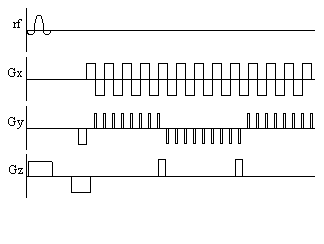##### Figure 2.18. Pulse sequence diagram for Echo Volumar Imaging

Often the main limitation in implementing fast imaging sequences such as EPI is switching the gradients at the fast rates required. A sequence which is similar to EPI, but slightly easier to implement is spiral imaging. This covers k-space in a spiral from the centre outwards, which requires sinusoidal gradients in x and y, increasing in amplitude with time. Such gradient waveforms are easier to produce than the gradients required for EPI. Spiral imaging also has the advantage of sampling the centre of k-space first, and so the low spatial frequencies, that affect the image the most are sampled first, when the signal has not been eroded by T2*. The pulse sequence diagram and coverage of k-space for spiral imaging are shown in Figure 2.19.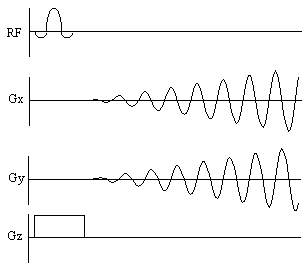##### Figure 2.19 Pulse sequence diagram and k-space sampling diagram for spiral imaging

In general imaging, the chemical shifts of the protons are ignored, and usually seen only as an artefact. However it is possible to image the chemical shifts, which gives not only spatial information but also spectral information. The technique, called Chemical Shift Imaging (CSI) , treats the chemical shift as an extra imaging gradient in the fourth dimension. By introducing a variable delay between the excitation pulse and imaging gradients, the chemical shift 'gradient' will phase encode in this direction. Fourier transformation in this case gives the conventional NMR spectrum.

Finally, it is possible to image nuclei other than the proton. Sodium, phosphorus and carbon-13 have all been used to form biomedical images. In the case of 13C, its low natural abundance makes it useful for tracer studies.